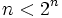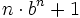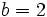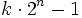# Cullen number

This article defines a property that can be evaluated for a natural number, i.e., every natural number either satisfies the property or does not satisfy the property.
View a complete list of properties of natural numbers

## Definition

Supposeis a natural number. The Cullen number parametrized by, denoted, is defined as:A Cullen number that is a prime is termed a Cullen prime.

## Relation with other properties

### Weaker properties

• Proth number: A Proth number is a number of the form,. Sincefor all, any Cullen number is a Proth number.
• Generalized Cullen number: A Cullen number of the formwhere. Note that the special case wheregives Cullen numbers.

### Other related properties

• Fermat number is a number of the form, and is also a special case of a Proth number.
• Sierpinski number is a number of the form, with.